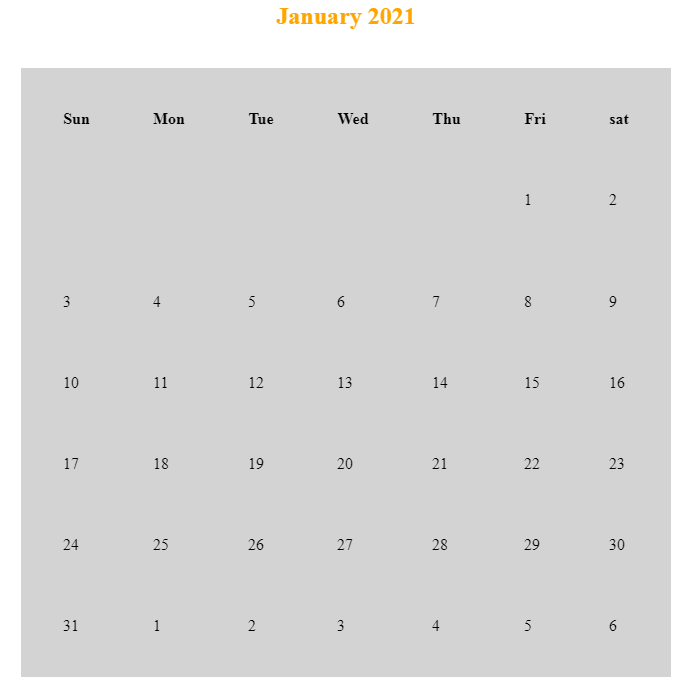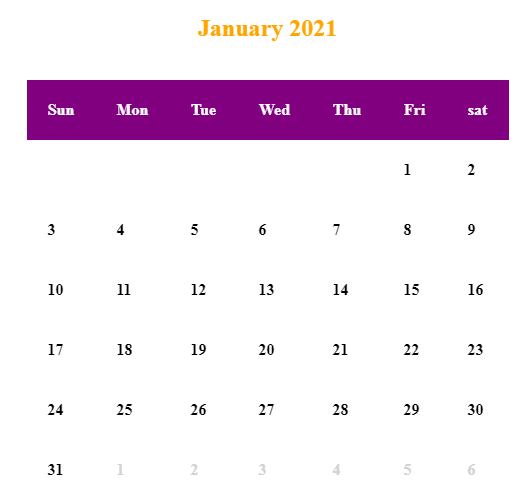Related Articles
Design a calendar using HTML and CSS
• Last Updated : 27 Jan, 2021

In this article, we will create a calendar using HTML, and CSS. First, we have to know a little about HTML. If someone does not know HTML and CSS, they will not be able to make the calendar better. The main focus of this article is on HTML tags and the way we are going to use CSS.

Approach: First we will be using the table tag, which will be used to create the structure of the calendar. This will give us an idea of how the calendar is created using HTML. Later we will apply some CSS property to make the design of the calendar better.

Creating Structure: In this section, we will create first the structure of the calendar by using the <table> tag. So this will help us to get the structure of the calendar.

## HTML

 ```<``html``>`` ` `<``body``>``    ```` ` `    ```` ` `    ````    ``<``h2` `align``=``"center"` `style``=``"color: orange;"``>``        ``January 2021``    ````    ``<``br` `/>``     ` `    ``<``table` `bgcolor``=``"lightgrey"` `align``=``"center"` `        ``cellspacing``=``"21"` `cellpadding``=``"21"``>``         ` `        ```` ` `        ```` ` `        ``<``caption` `align``=``"top"``>``            ````        ```` ` `        ```` ` `        ```` ` `        ``<``thead``>``            ``<``tr``>``                ````                ``<``th``>Sun``                ``<``th``>Mon``                ``<``th``>Tue``                ``<``th``>Wed``                ``<``th``>Thu``                ``<``th``>Fri``                ``<``th``>sat``            ````        ````         ` `        ``<``tbody``>``            ``<``tr``>``                ``<``td``>``                ``<``td``>``                ``<``td``>``                ``<``td``>``                ``<``td``>``                ``<``td``>1``                ``<``td``>2``            ````            ``<``tr``>``            ``<``tr``>``                ``<``td``>3``                ``<``td``>4``                ``<``td``>5``                ``<``td``>6``                ``<``td``>7``                ``<``td``>8``                ``<``td``>9``            ````            ``<``tr``>``                ``<``td``>10``                ``<``td``>11``                ``<``td``>12``                ``<``td``>13``                ``<``td``>14``                ``<``td``>15``                ``<``td``>16``            ````            ``<``tr``>``                ``<``td``>17``                ``<``td``>18``                ``<``td``>19``                ``<``td``>20``                ``<``td``>21``                ``<``td``>22``                ``<``td``>23``            ````            ``<``tr``>``                ``<``td``>24``                ``<``td``>25``                ``<``td``>26``                ``<``td``>27``                ``<``td``>28``                ``<``td``>29``                ``<``td``>30``            ````            ``<``tr``>``                ``<``td``>31``                ``<``td``>1``                ``<``td``>2``                ``<``td``>3``                ``<``td``>4``                ``<``td``>5``                ``<``td``>6``            ````        ````    `````` ` ``

Output:CSS Design and its attributes: We will use some CSS properties and attributes of the table tag to design the calendar. The attributes that we are going to use is the border and cellspacing and cellpadding. Here we have used an interesting property of CSS that is border-collapse. The purpose of the border-collapse is to make all the border to be collapsed into a single border.  Here we have also used the inline style attribute to make the dates of February be little visible.

CSS code:

```table {
border-collapse: collapse;
background: white;
color: black;
}
th, td {
font-weight: bold;
}
```

Attributes that we will use in the table tag:

```<table bgcolor="lightgrey" align="center"
cellspacing="21" cellpadding="21">  ```

Final code: This is the combination of all the above codes

## HTML

 ```<``html``>`` ` `<``head``>``    ``<``style``>``        ``table {``            ``border-collapse: collapse;``            ``background: white;``            ``color: black;``        ``}``         ` `        ``th,``        ``td {``            ``font-weight: bold;``        ``}``    `````` ` `<``body``>``    ```` ` `    ```` ` `    ````    ``<``h2` `align``=``"center"` `style``=``"color: orange;"``>``        ``January 2021``    ````    ``<``br` `/>`` ` `    ``<``table` `bgcolor``=``"lightgrey"` `align``=``"center"` `        ``cellspacing``=``"21"` `cellpadding``=``"21"``>`` ` `        ```` ` `        ```` ` `        ``<``caption` `align``=``"top"``>``            ````        ```` ` `        ```` ` `        ```` ` `        ``<``thead``>``            ``<``tr``>``                ````                ``<``th` `style``=``"color: white; background: purple;"``>``                    ``Sun``                ``<``th` `style``=``"color: white; background: purple;"``>``                    ``Mon``                ``<``th` `style``=``"color: white; background: purple;"``>``                    ``Tue``                ``<``th` `style``=``"color: white; background: purple;"``>``                    ``Wed``                ``<``th` `style``=``"color: white; background: purple;"``>``                    ``Thu``                ``<``th` `style``=``"color: white; background: purple;"``>``                    ``Fri``                ``<``th` `style``=``"color: white; background: purple;"``>``                    ``Sat``            ````        ```` ` `        ``<``tbody``>``            ``<``tr``>``                ``<``td``>``                ``<``td``>``                ``<``td``>``                ``<``td``>``                ``<``td``>``                ``<``td``>1``                ``<``td``>2``            ````            ``<``tr``>``            ``<``tr``>``                ``<``td``>3``                ``<``td``>4``                ``<``td``>5``                ``<``td``>6``                ``<``td``>7``                ``<``td``>8``                ``<``td``>9``            ````            ``<``tr``>``                ``<``td``>10``                ``<``td``>11``                ``<``td``>12``                ``<``td``>13``                ``<``td``>14``                ``<``td``>15``                ``<``td``>16``            ````            ``<``tr``>``                ``<``td``>17``                ``<``td``>18``                ``<``td``>19``                ``<``td``>20``                ``<``td``>21``                ``<``td``>22``                ``<``td``>23``            ````            ``<``tr``>``                ``<``td``>24``                ``<``td``>25``                ``<``td``>26``                ``<``td``>27``                ``<``td``>28``                ``<``td``>29``                ``<``td``>30``            ````            ``<``tr``>``                ``<``td``>31``                ``<``td``>1``                ``<``td``>2``                ``<``td``>3``                ``<``td``>4``                ``<``td``>5``                ``<``td``>6``            ````        ````    `````` ` ``

Output:My Personal Notes arrow_drop_up